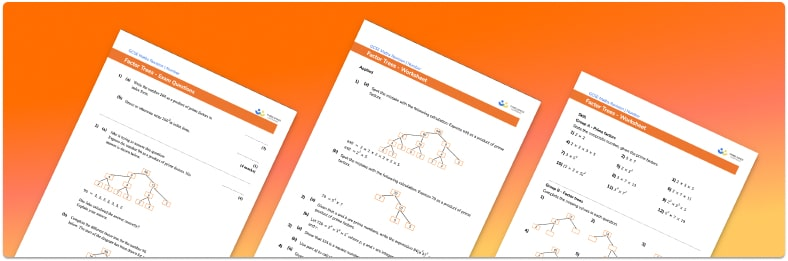# Factor Trees Worksheets• Section 1 of the factor trees worksheet contains 36 skills-based questions, in 3 groups to support differentiation
• Section 2 contains 4 applied factor trees questions with a mix of worded problems and deeper problem solving questions
• Section 3 contains 4 foundation and higher level GCSE exam style factor trees questions
• Answer keys and a mark scheme for all factor trees questions are provided
• Follows variation theory with plenty of opportunities for students to work independently at their own level
• All questions created by fully qualified expert secondary maths teachers
• Suitable for GCSE maths revision for AQA, OCR and Edexcel exam boards

• This field is for validation purposes and should be left unchanged.

You can unsubscribe at any time (each email we send will contain an easy way to unsubscribe). To find out more about how we use your data, see our privacy policy.

### Factor trees at a glance

Factor trees are used to find the prime factors of a number. Any number with two or more factors is called a composite number and can be written as a product of prime numbers.

To find the prime factors of a number we can use a prime factor tree with the given number at the top. We find a factor pair of the number and represent these on two branches. Next we find factor pairs of each of these numbers and continue in this way until we get to prime numbers. These prime numbers are the prime factors of the original number. We can write a number as a product of its prime factors by writing these prime factors in a product calculation, using exponents where appropriate.

Although multiple different prime factor trees could be created for a number, the final prime factorisation will always be the same. The prime factors of two or more numbers can be compared to find the lowest common multiple (LCM/least common multiple) or highest common factor (HCF/greatest common factor/GCF) of those numbers.

Looking forward, students can then progress to additional number worksheets, for example aorFor more teaching and learning support on Number our GCSE maths lessons provide step by step support for all GCSE maths concepts.

## Do you have KS4 students who need more focused attention to succeed at GCSE?There will be students in your class who require individual attention to help them succeed in their maths GCSEs. In a class of 30, it’s not always easy to provide.

Help your students feel confident with exam-style questions and the strategies they’ll need to answer them correctly with our dedicated GCSE maths revision programme.

Lessons are selected to provide support where each student needs it most, and specially-trained GCSE maths tutors adapt the pitch and pace of each lesson. This ensures a personalised revision programme that raises grades and boosts confidence.

Find out more